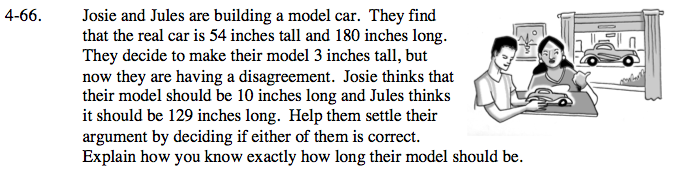### Home > CAAC > Chapter 4 > Lesson 4.1.7 > Problem4-66

4-66.Set up a proportion

Substitute in the given information.

Multiply both sides by x to get it in the numerator.

Multiply both sides by 180 to remove the fractions.

Solve for x.

Josie is correct.

$\frac{\text{car height}}{\text{car length}}=\frac{\text{ model height}}{\text{model length}}$

$\frac{54}{180}=\frac{3}{x}$

$x\left(\frac{54}{180}\right)=\left(\frac{3}{x}\right)x$

$\frac{54x}{180}=3$

$180\left(\frac{54x}{180}\right)=3(180)$

54x = 540

x = 10 inches